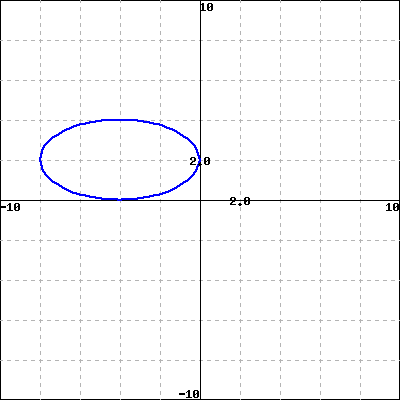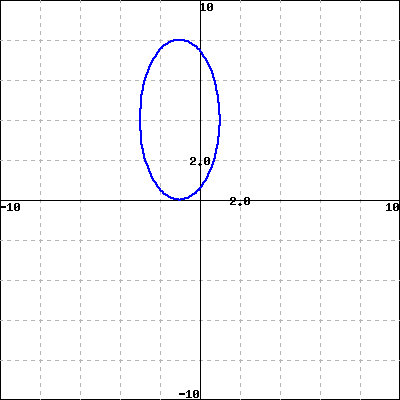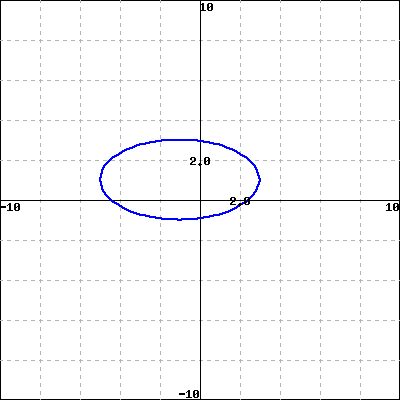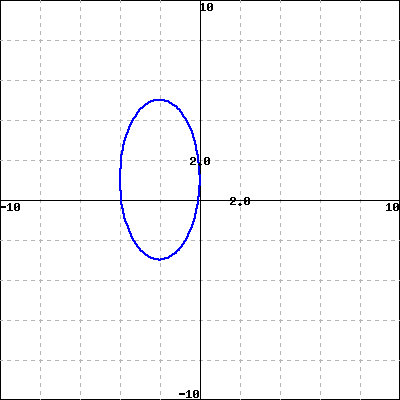A BC D

From the graphs above, select the graph of the unit circle stretched horizontally by a factor of 4, stretched vertically by a factor of 2, then shifted up by 1 units and left by 1 units:

Find an equation for the circle or ellipse described: